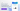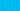Pricing# Accounting equation: a complete guide

Written by

No matter the size of a business, a basic accounting equation is used for formulating financial statements. This accounting equation is used around the world for a consistent international framework. So, what is the accounting equation, and what is its purpose?

## What is the accounting equation?

A double-entry accounting system is based on the accounting equation:

Total Assets = Liabilities + Equity

In this case, assets represent any of the company’s valuable resources, while liabilities are outstanding obligations. Combining liabilities and equity shows how the company’s assets are financed.

This basic equation offers a way for businesses to ensure that their financial statements are balanced. Any entries made on the debit side of a balance sheet should have a corresponding entry on the credit side.

## What is the purpose of the accounting equation?

To understand the purpose of the accounting equation, it’s first helpful to take a closer look at double-entry accounting. At the heart of this is the balance sheet, which shows a balance of total assets, total liabilities, and shareholder equity.

The accounting equation ensures that all uses of capital (assets) remain equal to all sources of capital (debt and equity).

Double-entry accounting requires that every business transaction be marked in at least two financial accounts. For example, if a business buys raw materials using cash, it would first mark this in the inventory accounts. The raw materials would be an asset, leading to an increase in inventory. The transaction should also be marked as a reduction of capital due to the spending of cash. According to double-entry accounting, this single transaction would require two separate accounting entries.

Similarly, when a company takes out a business loan, the borrowed money leads to an increase in assets. At the same time, this increases the company’s liability in the form of debt. As you can see from the examples above, double-entry accounting keeps the books balanced.

This is where the idea of the accounting equation comes in. The two sides of the equation must always add up to equal value. Assets must equal the sum of equity and liabilities.

By making this an international standard, it’s easier for global corporations to keep track of their accounts. It’s also helpful on a lower level by keeping all transactions in balance, with a verifiable relationship between each expense and its source of financing.

## Elements of accounting equation formula

There are three main elements of the accounting equation:

1. Assets

A company’s assets could include everything from cash to inventory. This consists of all equipment, prepaid expenses, receivables, and property – anything the business owns that reflects its value.

2. Liabilities

The second component of the accounting equation is liabilities. This category includes any obligations the company might have to third parties, such as accounts payable, deferred revenue, or other debts.

3. Equity

The final component is equity. This category includes the value of any investments made in the organisation, whether through the owners or shareholders. Owner’s equity will equal anything left from the assets after all liabilities have been paid.

These three elements of the accounting equation are what constitute a balance sheet. As a result, the equation is sometimes referred to as the balance sheet equation.

## How to balance the accounting equation

The equation provides an easy way for accounting teams to verify transactions. When determining how to balance the accounting equation, you must look at each of the three elements:

• Step 1: Locate the company’s total assets for the accounting period in question.

• Step 2: Add up all the liabilities from this same accounting period.

• Step 3: Locate the shareholder’s equity and add this figure to the liabilities.

• Step 4: Ensure that the total assets equal the sum of total equity and liabilities.

If the equation isn’t correct, this means it’s time to comb through the financial paperwork to find out if any transactions were recorded incorrectly.

You can also rearrange the equation to find out any of the missing parts. For example, suppose you know that Company A has total assets of \$10 million and equity of \$8 million. In that case, you can subtract the equity from assets to determine that the liabilities must total \$2 million. In this way, the accounting equation offers a simple standard for retaining balance.

## We can help

GoCardless helps you automate payment collection, cutting down on the amount of admin your team needs to deal with when chasing invoices. Find out how GoCardless can help you with ad hoc payments or recurring payments.